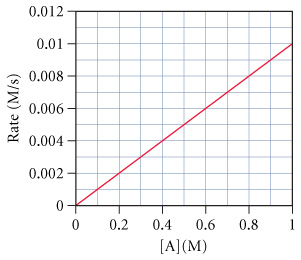# Problem: The graph below shows a plot of the rate of a reaction versus the concentration of the reactant A for the reaction A.What is the order of the reaction with respect to A?

###### FREE Expert Solution

Recall that the rate law only focuses on the reactant concentrations and has a general form of:

k = rate constant
X & Y = reactants
a & b = reactant orders

99% (228 ratings)###### Problem Details

The graph below shows a plot of the rate of a reaction versus the concentration of the reactant A for the reaction A.

What is the order of the reaction with respect to A?

Frequently Asked Questions

What scientific concept do you need to know in order to solve this problem?

Our tutors have indicated that to solve this problem you will need to apply the Rate Law concept. You can view video lessons to learn Rate Law. Or if you need more Rate Law practice, you can also practice Rate Law practice problems.

What professor is this problem relevant for?

Based on our data, we think this problem is relevant for Professor Laskin & Skrynnikov's class at PURDUE.